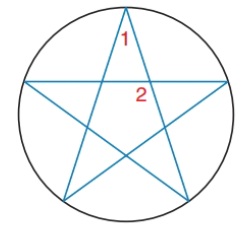Chapter 6.2, Problem 29EElementary Geometry For College St...

7th Edition
Alexander + 2 others
ISBN: 9781337614085

Solutions

Chapter
SectionElementary Geometry For College St...

7th Edition
Alexander + 2 others
ISBN: 9781337614085
Textbook Problem

For the five-pointed star (a regular pentagram) inscribed in the circle, find the measure of ∠ 1   and   ∠ 2 .To determine

To find:

The measure of 1and2.

Explanation

Given:

Calculation:

If 1 has the vertex on the circle:

mA=m1=mCD2=360°52=36°

If 2 has the vertex inside the circle.

m2=mAB+mCDE2=m

Still sussing out bartleby?

Check out a sample textbook solution.

See a sample solution

The Solution to Your Study Problems

Bartleby provides explanations to thousands of textbook problems written by our experts, many with advanced degrees!

Get Started

Convert the expressions in Exercises 6584 to power form. 4x23+x3/2623x2

Finite Mathematics and Applied Calculus (MindTap Course List)

In Exercises 1 and 2, simplify the expression. 4(10)75

Calculus: An Applied Approach (MindTap Course List)

Let g be the function defined by g(x) = x2 + 2x. Find g(a + h), g(a), g(a), a + g(a), and 1g(a).

Applied Calculus for the Managerial, Life, and Social Sciences: A Brief Approach

Find if , y = sin 2t.

Study Guide for Stewart's Multivariable Calculus, 8th

Let and g(x) = x + 3. Then (g ∘ h)(x) = ______.

Study Guide for Stewart's Single Variable Calculus: Early Transcendentals, 8th